# Chemical Reactions and Balancing Chemical Equations Chemical Reactions

• Slides: 47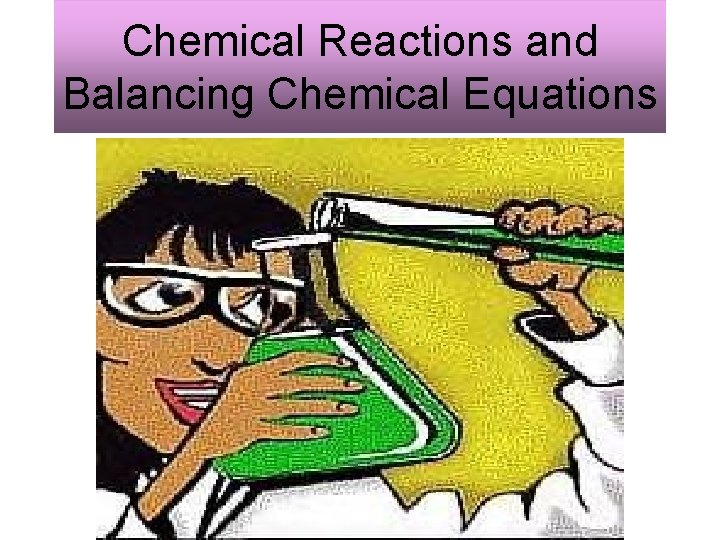Chemical Reactions and Balancing Chemical EquationsChemical Reactions Chemical Reaction: • The process in which substance undergo chemical changes that result in the formation of new substances.How to detect a chemical reaction There are 3 ways to detect that a chemical reaction has occurred: 1. New substances are formed. 2. Changes in properties such as a. Change in color b. Precipitate forms (when 2 solutions form a solid) c. A gas is producedHow to detect a chemical reaction • Gas forming Precipitate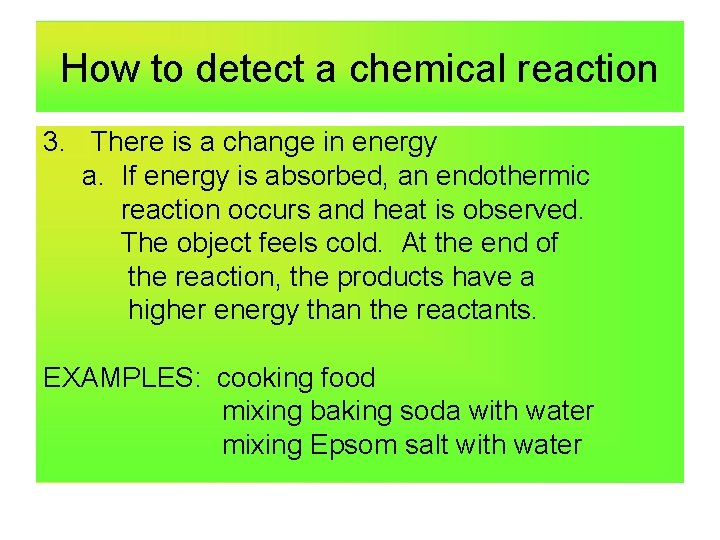How to detect a chemical reaction 3. There is a change in energy a. If energy is absorbed, an endothermic reaction occurs and heat is observed. The object feels cold. At the end of the reaction, the products have a higher energy than the reactants. EXAMPLES: cooking food mixing baking soda with water mixing Epsom salt with water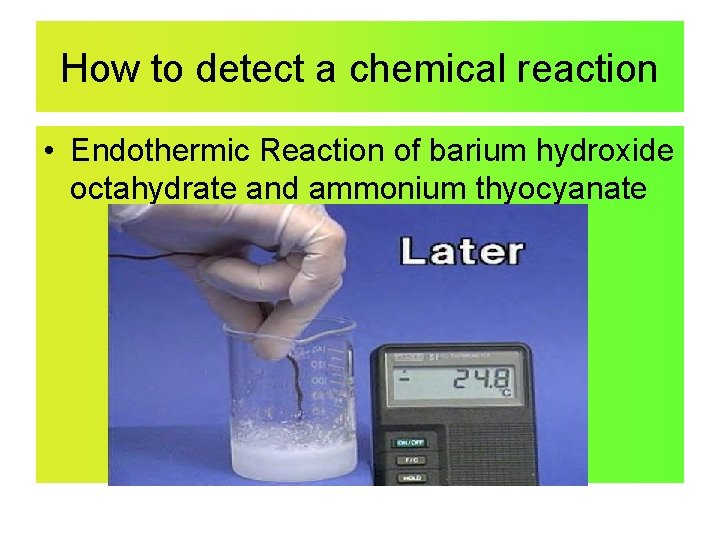How to detect a chemical reaction • Endothermic Reaction of barium hydroxide octahydrate and ammonium thyocyanate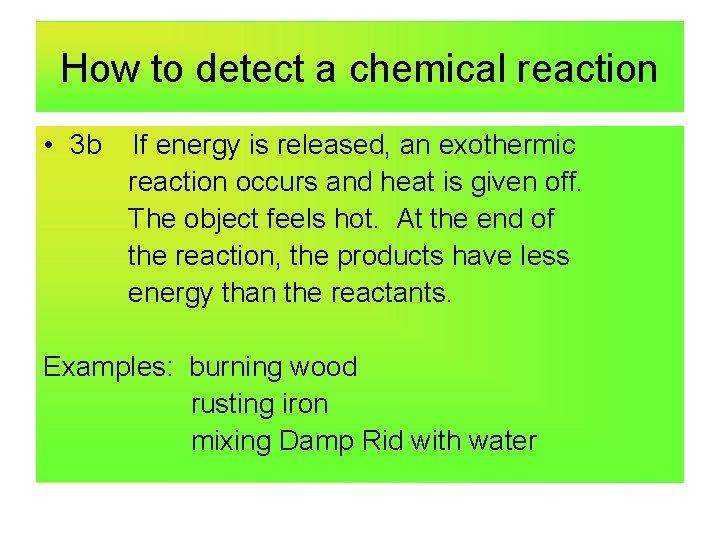How to detect a chemical reaction • 3 b If energy is released, an exothermic reaction occurs and heat is given off. The object feels hot. At the end of the reaction, the products have less energy than the reactants. Examples: burning wood rusting iron mixing Damp Rid with waterHow to detect a chemical reaction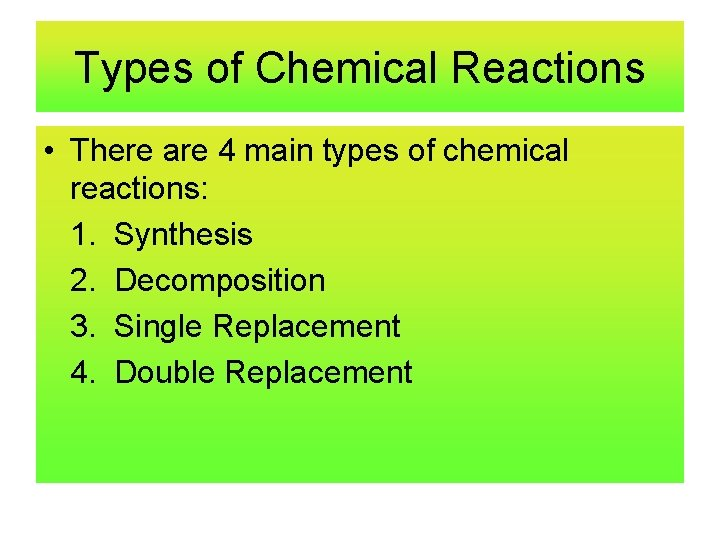Types of Chemical Reactions • There are 4 main types of chemical reactions: 1. Synthesis 2. Decomposition 3. Single Replacement 4. Double ReplacementMiddle School Dance Situation 1 SYNTHESIS REACTION Lab In a synthesis reaction two or more simple substances combine to form a more complex substance. Two or more reactants yielding one product is another way to identify a synthesis reaction. Example: H 2 + O -- H 2 O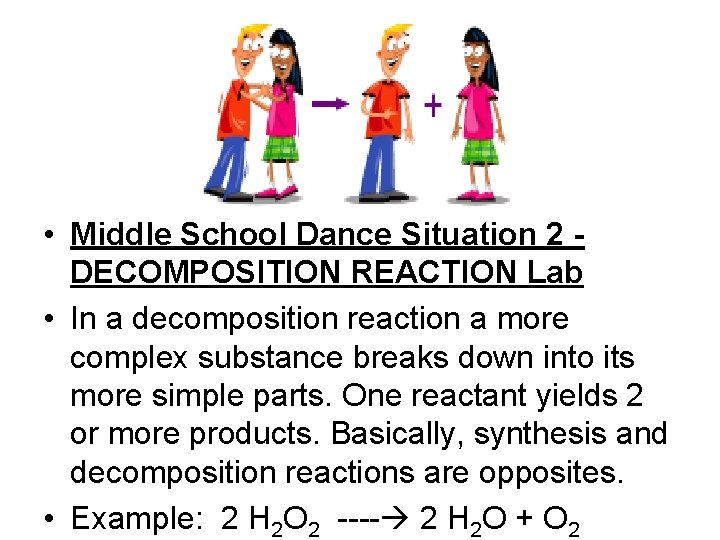• Middle School Dance Situation 2 DECOMPOSITION REACTION Lab • In a decomposition reaction a more complex substance breaks down into its more simple parts. One reactant yields 2 or more products. Basically, synthesis and decomposition reactions are opposites. • Example: 2 H 2 O 2 ---- 2 H 2 O + O 2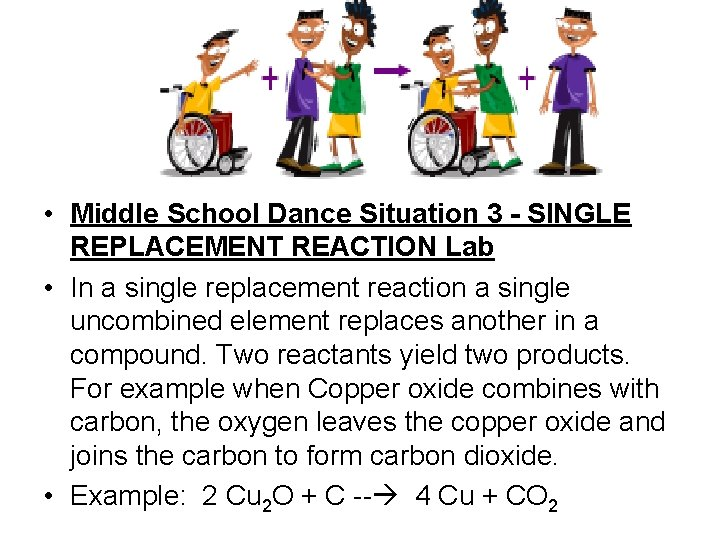• Middle School Dance Situation 3 - SINGLE REPLACEMENT REACTION Lab • In a single replacement reaction a single uncombined element replaces another in a compound. Two reactants yield two products. For example when Copper oxide combines with carbon, the oxygen leaves the copper oxide and joins the carbon to form carbon dioxide. • Example: 2 Cu 2 O + C -- 4 Cu + CO 2• Middle School Dance Situation 4 - DOUBLE REPLACEMENT REACTION Lab • In a double replacement reaction parts of two compounds switch places to form two new compounds. Two reactants yield two products. For example when Iron Sulfuide combines with hydrochloric acid, two new compounds—iron chloride and hyrogen sulfide are formed because the sodium and chlorine switched places. • Example: Fe. S + 2 HCl ---- Fe. Cl 2 + H 2 SChemical Equations Chemical Equation A way to show a chemical reaction using symbols and chemical formulas instead of words. Reactants: The substances you start with Products: The substances created from the reactionChemical Equations • EXAMPLE • H 2 + O Reactants ---- H 2 O ProductsChemical Equations • All chemical reactions follow the Law of Conservation of Mass which states that in a chemical reaction, the total mass of the reactants must equal the total mass of the products.Balancing Chemical Equations Since all chemical reactions follow the Law of Conservation of Mass, a chemical equation must be balanced. This means that the total # and kinds of atoms on the reactant side must be equal to the total # and kinds of atoms on the product side. The mass before the reaction must be equal to the mass after the reaction.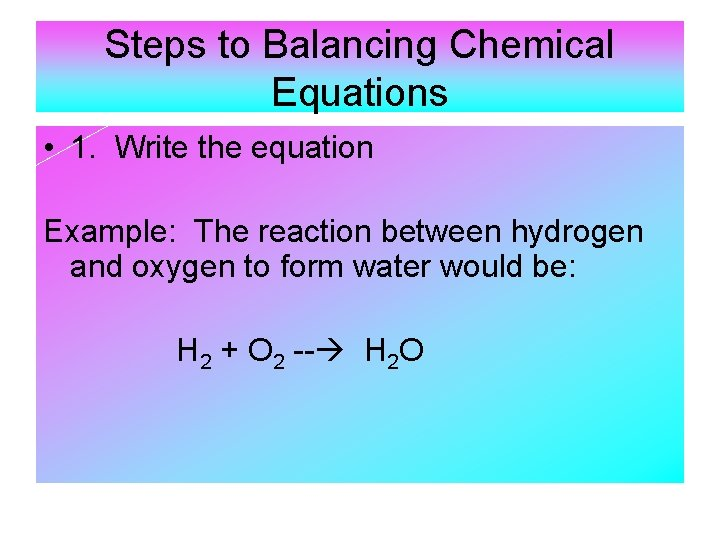Steps to Balancing Chemical Equations • 1. Write the equation Example: The reaction between hydrogen and oxygen to form water would be: H 2 + O 2 -- H 2 O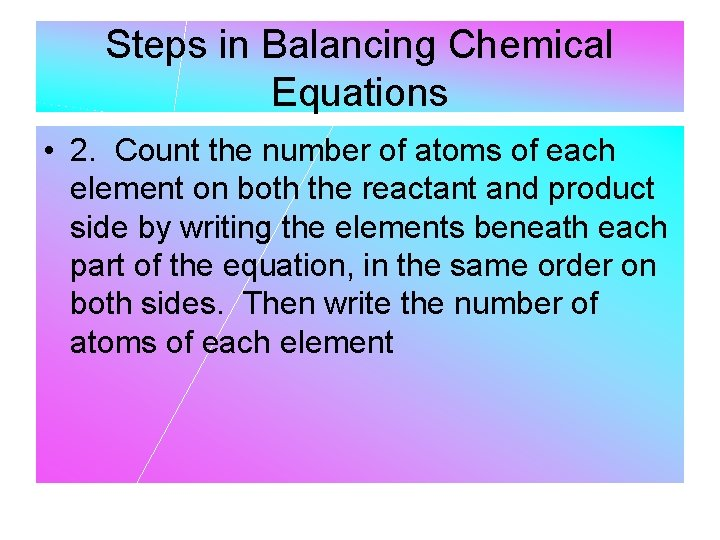Steps in Balancing Chemical Equations • 2. Count the number of atoms of each element on both the reactant and product side by writing the elements beneath each part of the equation, in the same order on both sides. Then write the number of atoms of each elementSteps in Balancing chemical equations • EXAMPLE H 2 + O 2 H=2 O=2 ---- H 2 O H=2 O=1 You can see that the equation is not balanced because there are 2 atoms of oxygen on the reactant side and only 1 on the product sideSteps to Balancing chemical equations • 3. Use coefficients to balance the atoms. Each side must have exactly the same kind and same number of atoms. • Coefficient: A number placed “ IN FRONT” of a chemical formula in an equation. Example: 2 H 2 O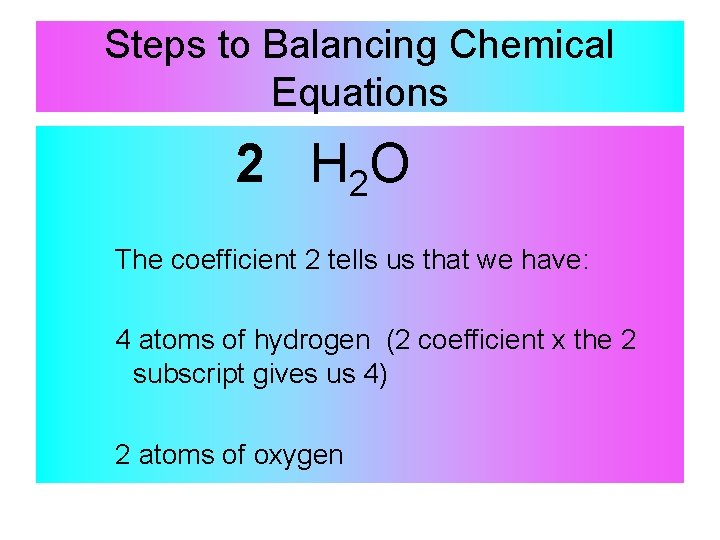Steps to Balancing Chemical Equations 2 H 2 O The coefficient 2 tells us that we have: 4 atoms of hydrogen (2 coefficient x the 2 subscript gives us 4) 2 atoms of oxygenSteps to Balancing Chemical Equations • You CAN change coefficients. For example: if you needed 8 hydrogen atoms and 4 oxygen atoms to balance, simply change the 2 coefficient to a 4. 2 H 2 O = 4 Hydrogen and 2 oxygen 4 H 2 O = 8 Hydrogen and 4 oxygen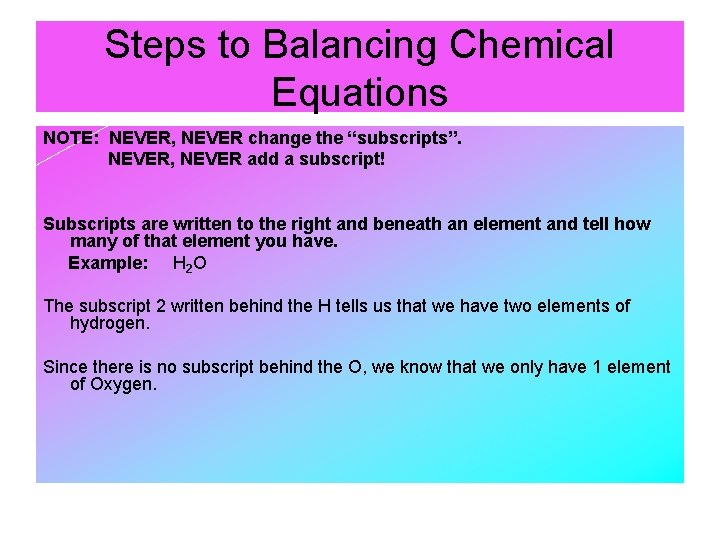Steps to Balancing Chemical Equations NOTE: NEVER, NEVER change the “subscripts”. NEVER, NEVER add a subscript! Subscripts are written to the right and beneath an element and tell how many of that element you have. Example: H 2 O The subscript 2 written behind the H tells us that we have two elements of hydrogen. Since there is no subscript behind the O, we know that we only have 1 element of Oxygen.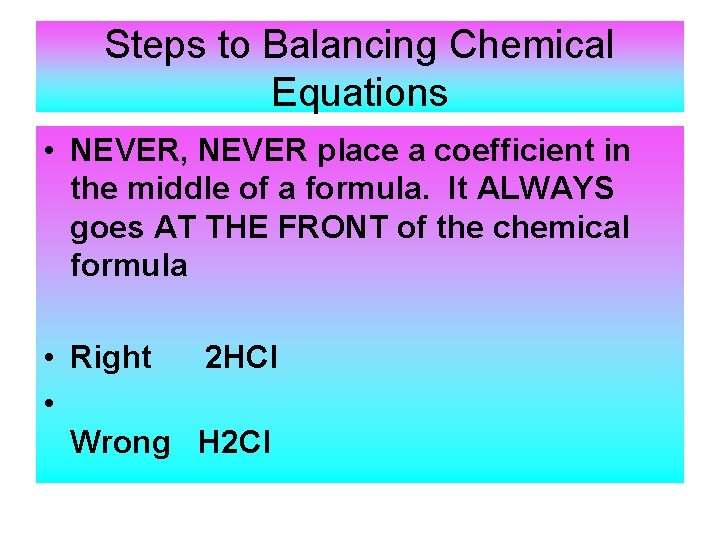Steps to Balancing Chemical Equations • NEVER, NEVER place a coefficient in the middle of a formula. It ALWAYS goes AT THE FRONT of the chemical formula • Right 2 HCl • Wrong H 2 ClSteps to Balancing A Chemical Equation • If two or more elements are enclosed in parentheses with a subscript behind the parenthesis, the subscript applies to every element INSIDE the parentheses. • Example: C(OH)2 The subscript 2 behind the parentheses tells us that we have 2 elements of Oxygen and 2 elements of Hydrogen. Note that the 2 behind the parenthesis DOES NOT apply to the Carbon since it is not enclosed in the parentheses.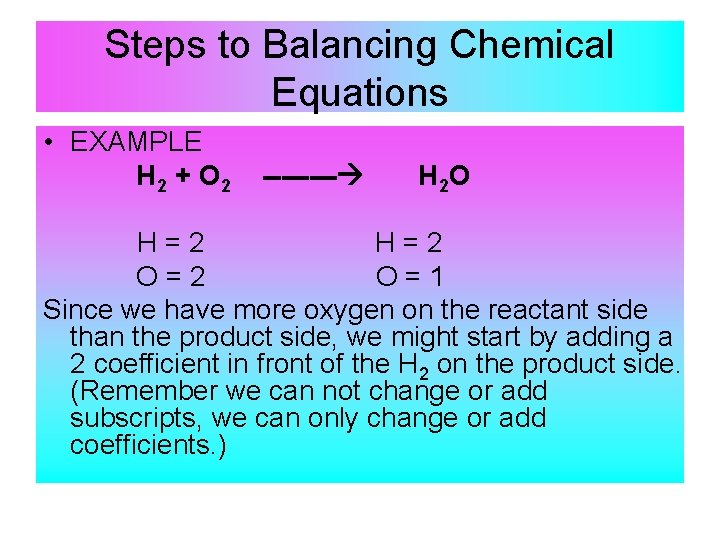Steps to Balancing Chemical Equations • EXAMPLE H 2 + O 2 ---- H 2 O H=2 O=2 O=1 Since we have more oxygen on the reactant side than the product side, we might start by adding a 2 coefficient in front of the H 2 on the product side. (Remember we can not change or add subscripts, we can only change or add coefficients. )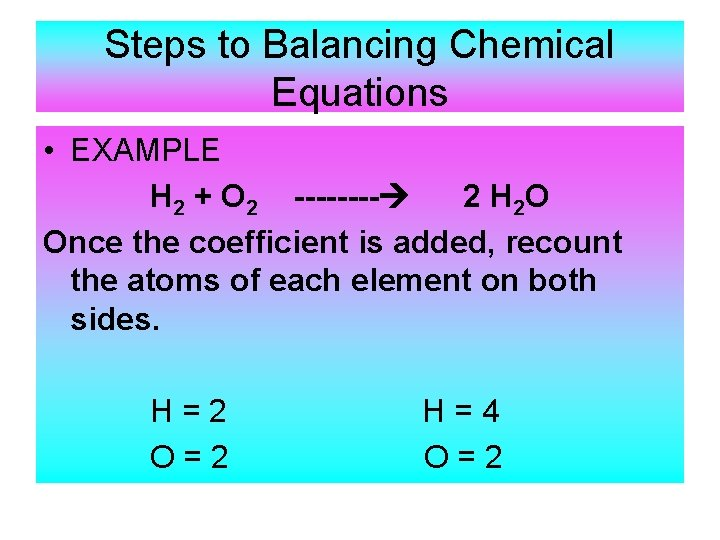Steps to Balancing Chemical Equations • EXAMPLE H 2 + O 2 ---- 2 H 2 O Once the coefficient is added, recount the atoms of each element on both sides. H=2 O=2 H=4 O=2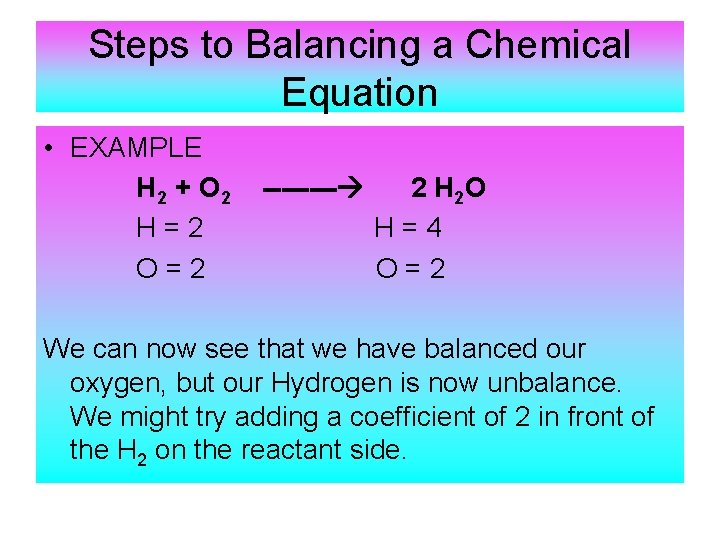Steps to Balancing a Chemical Equation • EXAMPLE H 2 + O 2 H=2 O=2 ---- 2 H 2 O H=4 O=2 We can now see that we have balanced our oxygen, but our Hydrogen is now unbalance. We might try adding a coefficient of 2 in front of the H 2 on the reactant side.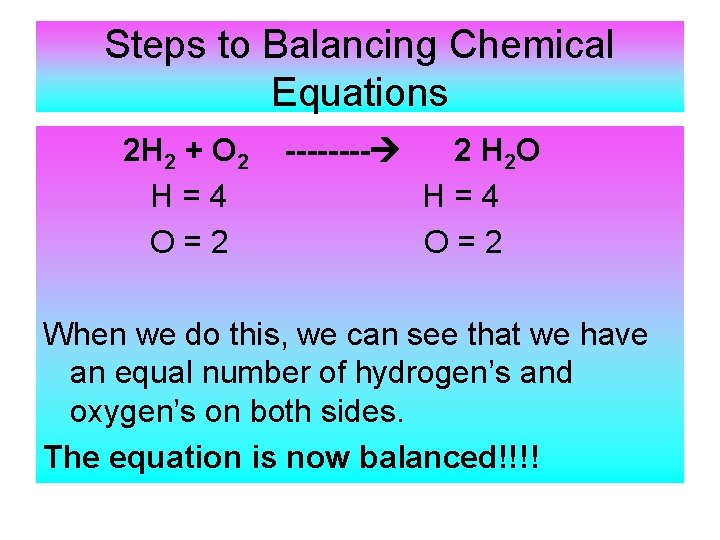Steps to Balancing Chemical Equations 2 H 2 + O 2 H=4 O=2 ---- 2 H 2 O H=4 O=2 When we do this, we can see that we have an equal number of hydrogen’s and oxygen’s on both sides. The equation is now balanced!!!!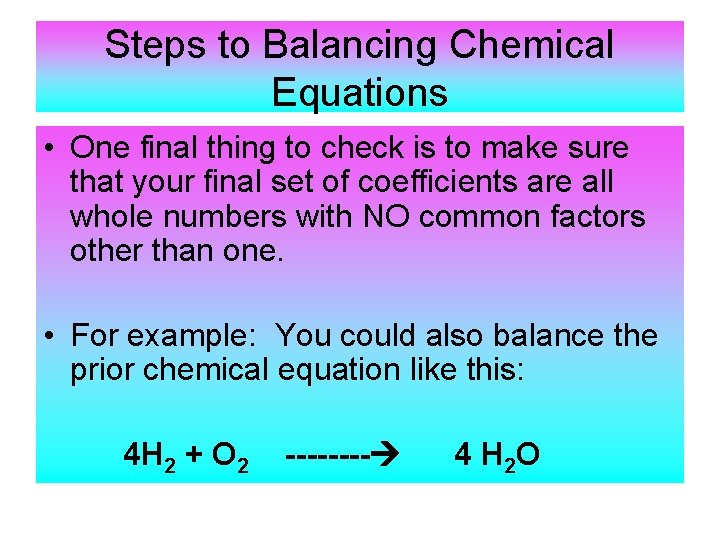Steps to Balancing Chemical Equations • One final thing to check is to make sure that your final set of coefficients are all whole numbers with NO common factors other than one. • For example: You could also balance the prior chemical equation like this: 4 H 2 + O 2 ---- 4 H 2 O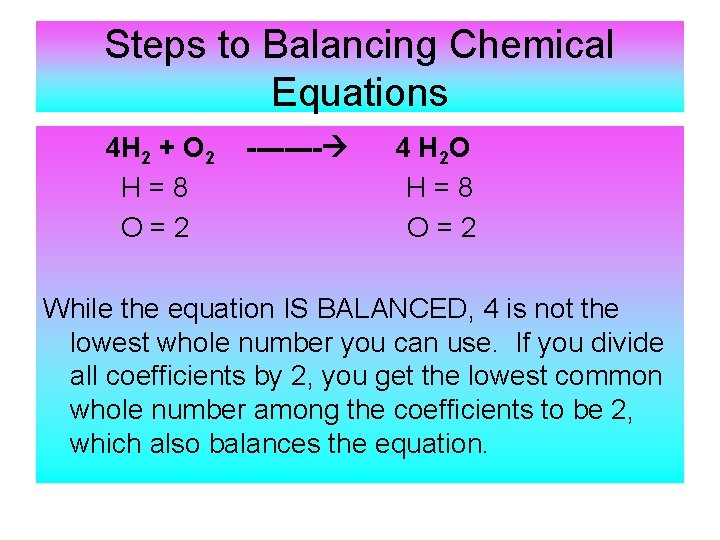Steps to Balancing Chemical Equations 4 H 2 + O 2 H=8 O=2 ---- 4 H 2 O H=8 O=2 While the equation IS BALANCED, 4 is not the lowest whole number you can use. If you divide all coefficients by 2, you get the lowest common whole number among the coefficients to be 2, which also balances the equation.Example 2 Mg + O 2 ---- Mg. O 1. Write Equation 2. Count Atoms Mg + O 2 Mg = 1 O=2 ---- Mg. O Mg = 1 O=1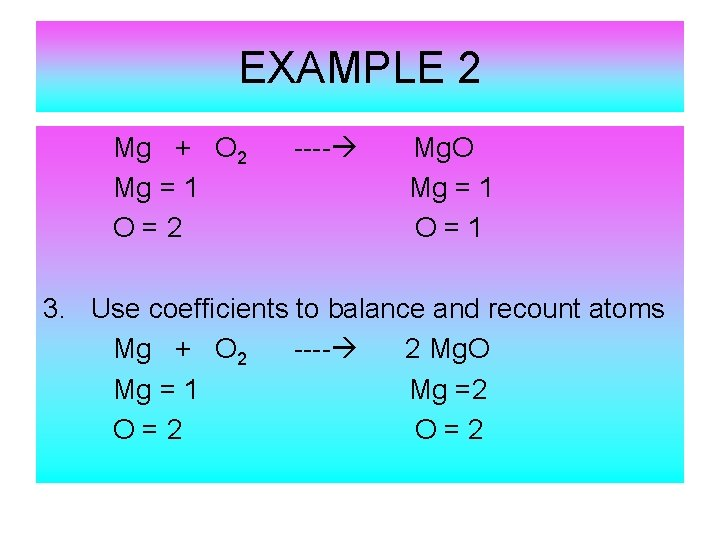EXAMPLE 2 Mg + O 2 Mg = 1 O=2 ---- Mg. O Mg = 1 O=1 3. Use coefficients to balance and recount atoms Mg + O 2 ---- 2 Mg. O Mg = 1 Mg =2 O=2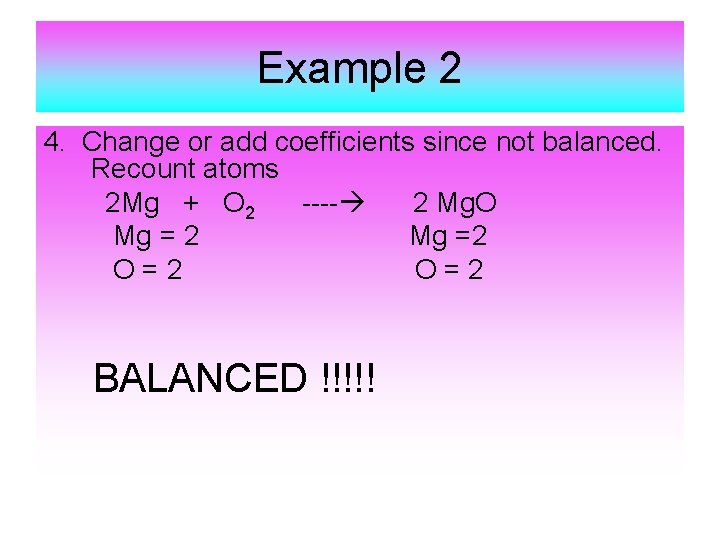Example 2 4. Change or add coefficients since not balanced. Recount atoms 2 Mg + O 2 ---- 2 Mg. O Mg = 2 Mg =2 O=2 BALANCED !!!!!Start a Reaction • A chemical reaction will not occur if the reactants DO NOT have enough energy to break their chemical bonds. • The minimum amount of energy needed to start a chemical reaction is called ACTIVATION ENERGY.Starting a Reaction • Rates of Chemical Reaction Chemists can speed up or slow down chemical reactions by changing factors such as: 1. Surface area 2. Temperature 3. Concentration 4. Using a catalyst 5. Using an inhibitor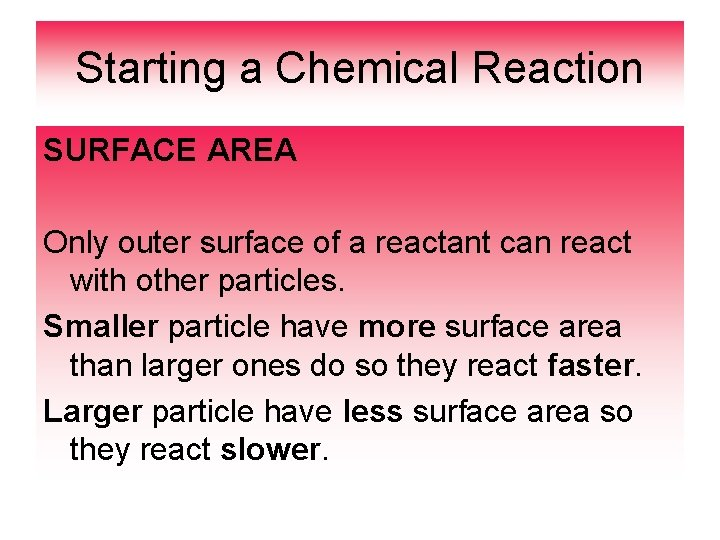Starting a Chemical Reaction SURFACE AREA Only outer surface of a reactant can react with other particles. Smaller particle have more surface area than larger ones do so they react faster. Larger particle have less surface area so they react slower.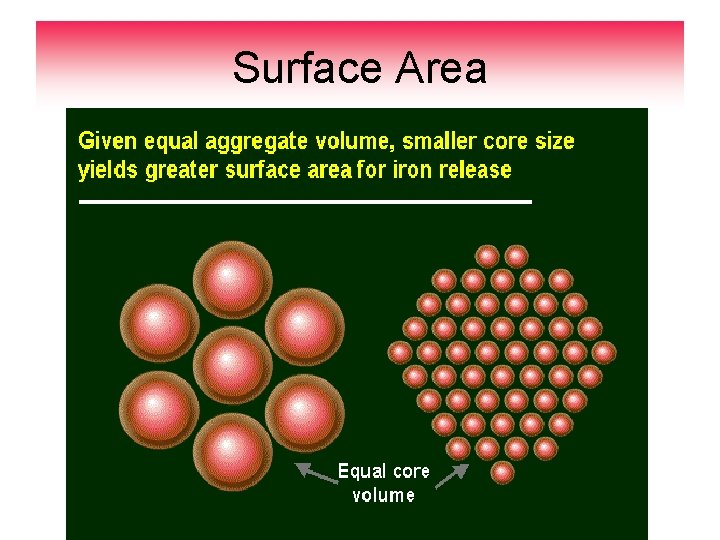Surface Area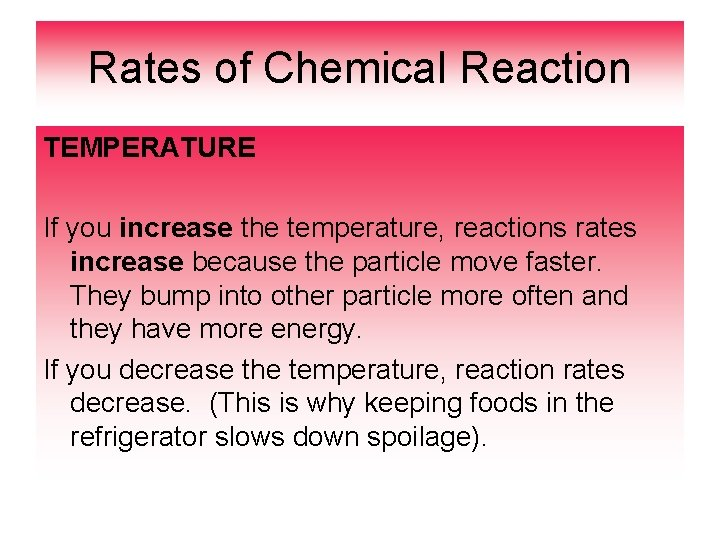Rates of Chemical Reaction TEMPERATURE If you increase the temperature, reactions rates increase because the particle move faster. They bump into other particle more often and they have more energy. If you decrease the temperature, reaction rates decrease. (This is why keeping foods in the refrigerator slows down spoilage).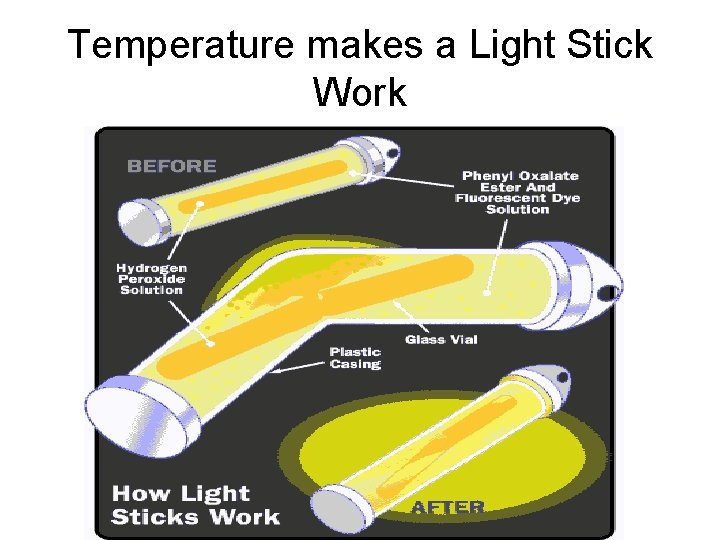Temperature makes a Light Stick WorkRates of Chemical Reactions CONCENTRATION Concentration is the amount of substance in a given volume. Increasing concentration supplies more particle to react so reaction occurs faster: Increase concentration = speedy reaction Decrease concentration = slower reaction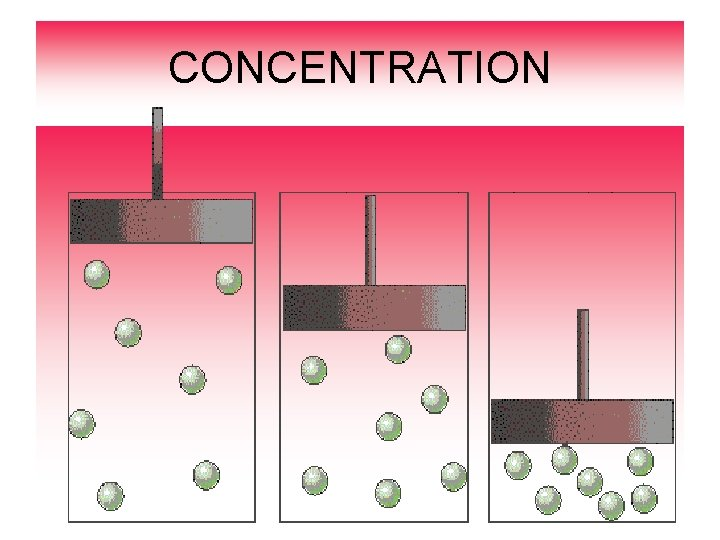CONCENTRATION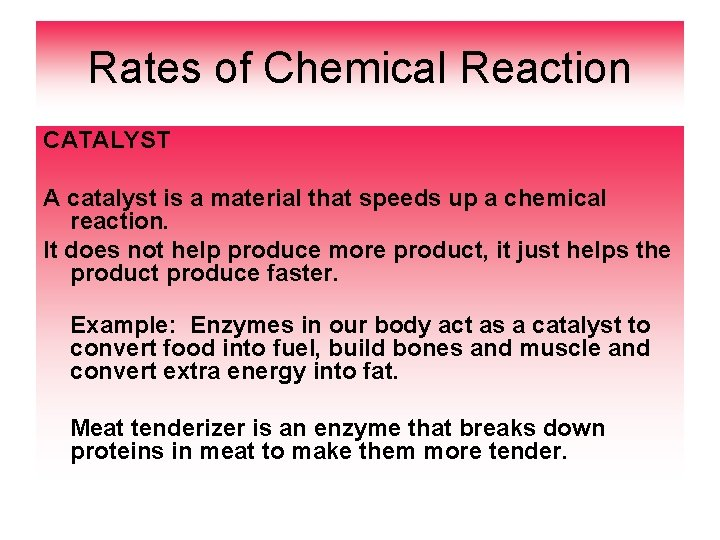Rates of Chemical Reaction CATALYST A catalyst is a material that speeds up a chemical reaction. It does not help produce more product, it just helps the product produce faster. Example: Enzymes in our body act as a catalyst to convert food into fuel, build bones and muscle and convert extra energy into fat. Meat tenderizer is an enzyme that breaks down proteins in meat to make them more tender.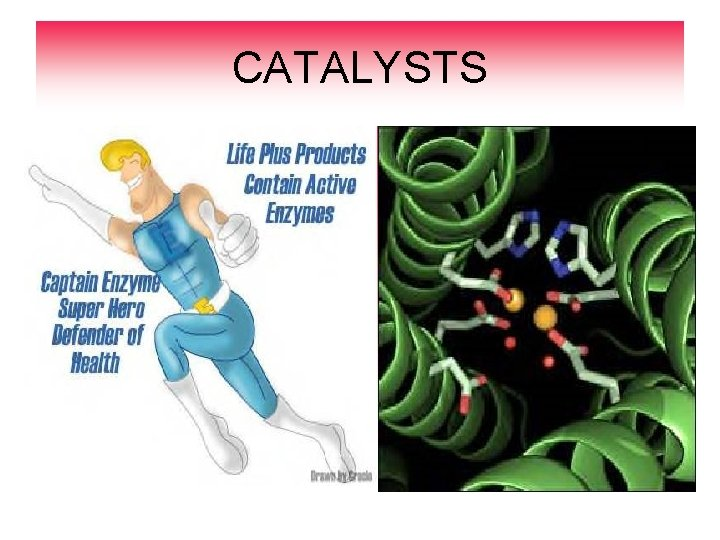CATALYSTS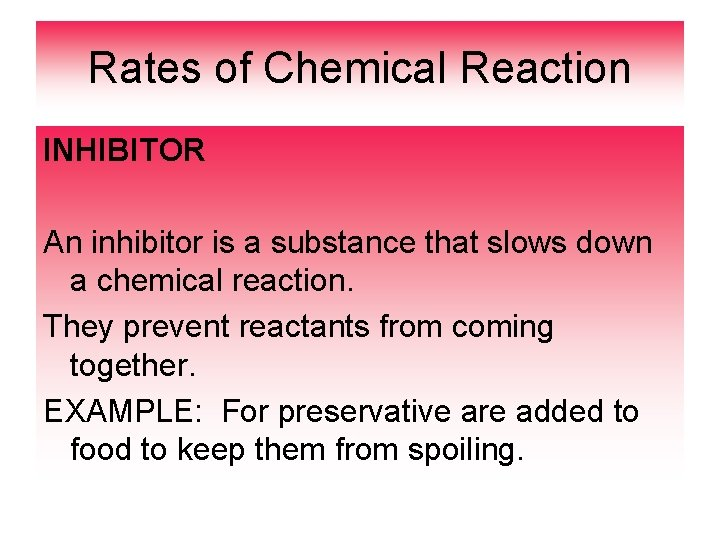Rates of Chemical Reaction INHIBITOR An inhibitor is a substance that slows down a chemical reaction. They prevent reactants from coming together. EXAMPLE: For preservative are added to food to keep them from spoiling.INHIBITORS